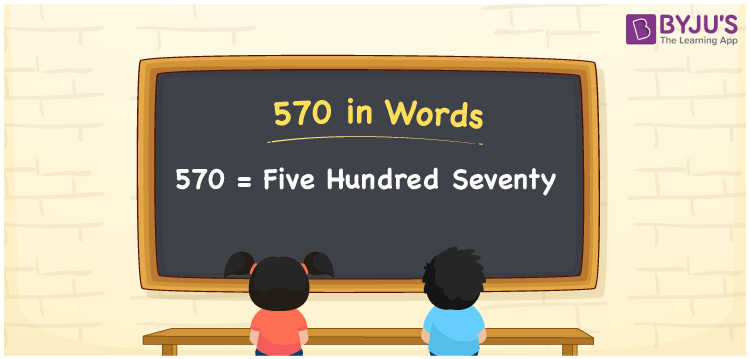# 570 in Words

570 in words is written as Five hundred seventy. In both the International System of Numerals and the Indian System of Numerals, 570 is written as Five hundred seventy. The number 570 is a Cardinal Number as it represents some quantity. For example, the “cost of the book is 570 rupees”.

 570 in Words Five hundred seventy Five hundred seventy in Number 570

## 570 in English Words

We write 570 in English Words using the letters of the English alphabet. Therefore, we read 570 in English as “Five hundred seventy.”## How to Write 570 in Words?

To write 570 in words, we shall use the place value chart. In the place value chart, write 0 in the ones, 7 in the tens, and 5 in the hundreds. Now let us make a place value chart to write the number 570 in words.

 Hundreds Tens Ones 5 7 0

Thus, we can write the expanded form as

5 × Hundred + 7 × Ten + 0 × One

= 5 × 100 + 7 × 10 + 0 × 1

= 500 + 70 + 0

= 570

= Five Hundred seventy

570 is a natural number which is the successor of 569 and the predecessor of 571.

570 in words – Five Hundred seventy

• Is 570 an odd number? – No
• Is 570 an even number? – Yes
• Is 570 a perfect square number? – No
• Is 570 a perfect cube number? – No
• Is 570 a prime number? – No
• Is 570 a composite number? – Yes

## Frequently Asked Questions on 570 in Words

Q1

### How to write 570 in words?

570 in words is written as Five Hundred seventy.
Q2

### How to write 570 in the International and Indian System of Numerals?

In both, the system of numerals, 570 in words, is written as Five Hundred seventy.
Q3

### How to write 570 in a place value chart?

In the place value chart, write 0 in the ones, 7 in the tens, and 5 in the hundreds.